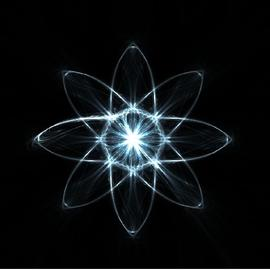# How to Make Infinite to be Finite

As I read the wiki entry on Transfinite number, some problems come to my mind.

## Take Finite out of Infinite

what is left after I take a finite subset out of an infinite set? Consider the Hilbert’s paradox of the Grand Hotel. If room 1 is out of services, then we can move the guest currently in room 1 to room 2, the guest currently in room 2 to room 3, and so on, moving every guest from his current room $n$ to room $n+1$. After this, room 1 is not needed. By repeating this procedure, it is possible to cancel any finite number of rooms which are out of services. The answer to the problem is, when we take a finite subset out of an infinite set, the remaining set is still infinite.

## Take Infinite out of Infinite

what is left after I take an infinite subset out of an infinite set?

### Case 1

Take the Hilbert’s paradox of the Grand Hotel as an example. If all rooms of odd number are out of services, then we can first assume only room 1 is not availabe, the guest currently in room 1 is moved to room 2, guest in room 2 to room 3, and so on, moving every guest from his current room $n$ to room $n+1$. After this, room 1 is not needed. By repeating this procedure, it is possible to cancel all odd rooms. In this case, the answer to the problem is, when we take an infinite subset (set of odd numbers) out of an infinite set, the remaining set is still infinite.

### Case 2

Take the set of natural numbers as example. We simply make the infinite subset $A$ be $A = {x \in N | x \neq 1 }$. It’s easy to see that A is infinite, and the complement set of $A$ over $N$ is of size 1. In this case, the answer to the problem is, when we take an infinite subset (set of $A$) out of an infinite set, the remaining set is finite.

Here is what confused me most. The cardinality of set of odd numbers and set $A$ are both Aleph-null, why their complements over $N$ are different? Currently, I make myself convinced that the problem occurs due to the ambiguity of complement over N.##### Kun WANG
###### Senior Researcher

My research focuses on characterizing, verifying, validating, mitigating, and correcting quantum errors inherent in quantum devices.